# Difference between revisions of "0708-1300/Class notes for Tuesday, November 13"

Announcements go here

## Typed Notes

The notes below are by the students and for the students. Hopefully they are useful, but they come with no guarantee of any kind.

### First Hour

We begin with a review of last class. Since no one has typed up the notes for last class yet, I will do the review here.

Recall had an association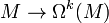$M\rightarrow\Omega^k(M)$ which was the "k forms on M" which equaled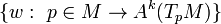$\{w:\ p\in M\rightarrow A^k(T_p M)\}$

where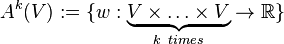$A^k(V):=\{w:\underbrace{V\times\ldots\times V}_{k\ times} \rightarrow\mathbb{R}\}$ which is

1) Multilinear

2) Alternating

We had proved that :

1)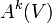$A^k(V)$ is a vector space

2) there was a wedge product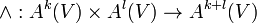$\wedge:A^k(V)\times A^l(V)\rightarrow A^{k+l}(V)$ via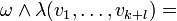$\omega\wedge\lambda(v_1,\ldots,v_{k+l}) =$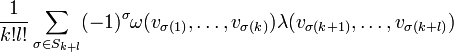$\frac{1}{k!l!}\sum_{\sigma\in S_{k+l}}(-1)^{\sigma} \omega(v_{\sigma(1)},\ldots,v_{\sigma(k)})\lambda(v_{\sigma(k+1)},\ldots,v_{\sigma(k+l)})$ that is

a) bilinear

b) associative

c) supercommutative, i.e.,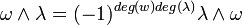$\omega\wedge\lambda = (-1)^{deg(w)deg(\lambda)}\lambda\wedge\omega$

From these definitions we can define for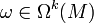$\omega\in\Omega^k(M)$ and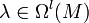$\lambda\in\Omega^l(M)$ that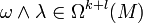$\omega\wedge\lambda\in\Omega^{k+l}(M)$ with the same properties as above.

Claim

If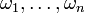$\omega_1,\ldots,\omega_n$ is a basis of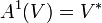$A^1(V) = V^*$ then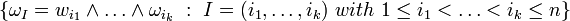$\{\omega_I = w_{i_1}\wedge\ldots\wedge\omega_{i_k}\ :\ I=(i_1,\ldots,i_k)\ with\ 1\leq i_1<\ldots is a basis of$A^k(V)$ and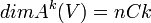$dim A^k(V) = nCk$

If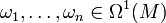$\omega_1,\ldots,\omega_n\in\Omega^1(M)$ and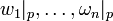$w_1 |_p,\ldots,\omega_n |_p$ a basis of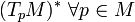$(T_p M)^*\ \forall p\in M$ then any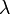$\lambda$ can be written as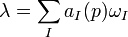$\lambda = \sum_I a_I(p)\omega_I$ where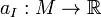$a_I:M\rightarrow\mathbb{R}$ are smooth.

The equivalence of these is left as an exercise.

Example

Let us investigate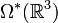$\Omega^*(\mathbb{R}^3)$ (the * just means "anything").

Now,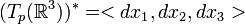$(T_p(\mathbb{R}^3))^* = $ where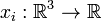$x_i:\mathbb{R}^3\rightarrow\mathbb{R}$ and so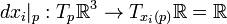$dx_i|_p:T_p\mathbb{R}^3\rightarrow T_{x_i(p)}\mathbb{R} =\mathbb{R}$

Hence,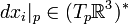$dx_i|_p\in (T_p\mathbb{R}^3)^*$

Now,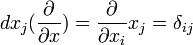$dx_j(\frac{\partial}{\partial x}) = \frac{\partial}{\partial x_i}x_j =\delta_{ij}$ and hence we get a basis.

So,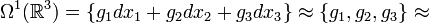$\Omega^1(\mathbb{R}^3) = \{g_1 dx_1 + g_2 dx_2 + g_3 dx_3\}\approx\{g_1,g_2,g_3\}\approx$ {vector fields on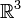$\mathbb{R}^3$}

where the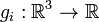$g_i:\mathbb{R}^3\rightarrow\mathbb{R}$ are smooth.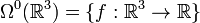$\Omega^0(\mathbb{R}^3) = \{f:\mathbb{R}^3\rightarrow\mathbb{R}\}$

This is because to each point p we associate something that takes zero copies of the tangent space into the real numbers. Thus to each p we associate a number.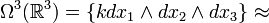$\Omega^3(\mathbb{R}^3) = \{kdx_1\wedge dx_2\wedge dx_3\} \approx$ {functions} where again the k is just a smooth function from$\mathbb{R}^3$ to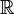$\mathbb{R}$.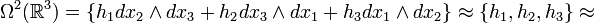$\Omega^2(\mathbb{R}^3) = \{h_1 dx_2\wedge dx_3 + h_2 dx_3\wedge dx_1 + h_3 dx_1\wedge dx_2\}\approx \{h_1,h_2,h_3\}\approx$ {vector fields}

Aside

Recall our earlier discussion of how points and things like points (curves, equivalence classes of curves) pushfoward while things dual to points (functions) pullback and that things dual to functions (such as derivations) push forward. See earlier for the precise definitions.

Now differential forms pull back, i.e., for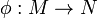$\phi:M\rightarrow N$ then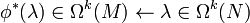$\phi^*(\lambda)\in\Omega^k(M)\leftarrow\lambda\in\Omega^k(N)$ via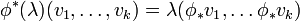$\phi^*(\lambda)(v_1,\ldots,v_k)=\lambda(\phi_* v_1,\ldots \phi_* v_k)$

The pullback preserves all the properties discussed above and is well defined. In particular, it is compatible with the wedge product via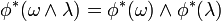$\phi^*(\omega\wedge\lambda)=\phi^*(\omega)\wedge\phi^*(\lambda)$

Theorem-Definition

Given M,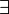$\exists$ ! linear map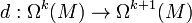$d:\Omega^k(M)\rightarrow\Omega^{k+1}(M)$ satisfies

1) If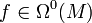$f\in\Omega^0(M)$ then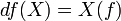$df(X) = X(f)$ for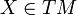$X\in TM$

2)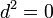$d^2 = 0$. I.e. if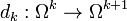$d_k:\Omega^k\rightarrow\Omega^{k+1}$ and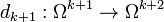$d_{k+1}:\Omega^{k+1}\rightarrow\Omega^{k+2}$ then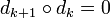$d_{k+1}\circ d_k = 0$.

3)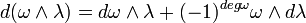$d(\omega\wedge\lambda) = d\omega\wedge\lambda + (-1)^{deg\omega}\omega\wedge d\lambda$

### Second Hour

Some notes about the above definition:

1) When we restrict our d to functions we just get the old meaning for d.

2) Philosophically, there is a duality between differential forms and manifolds and that duality is given by integration. In this duality, d is the adjoint of the boundary operator on manifolds. For manifolds, the boundary of the boundary is empty and hence it is reasonable that$d^2=0$ on differential forms.

3) To remember the formula in 3 given above and others like it, it helps to keep in mind what objects are "odd" and what are "even" and thus when commuting such operators we will get the signs as you would expect from multiplying objects that are either odd or even.

Example

Let us aim for a formula for d on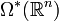$\Omega^*(\mathbb{R}^n)$.

Lets compute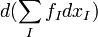$d(\sum_I f_I dx_I)$ where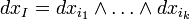$dx_I = dx_{i_1}\wedge\ldots\wedge dx_{i_k}$ and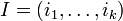$I = (i_1,\ldots,i_k)$

Then,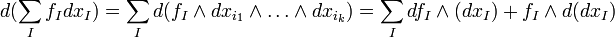$d(\sum_I f_I dx_I) = \sum_I d(f_I \wedge dx_{i_1}\wedge\ldots\wedge dx_{i_k}) = \sum_I df_I\wedge(dx_I) + f_I\wedge d(dx_I)$

The last term vanishes because of (2) in the theorem (proving uniqueness!)

Now, as an aside, we claim that for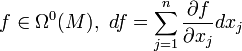$f\in\Omega^0(M),\ df = \sum_{j=1}^{n}\frac{\partial f}{\partial x_j} dx_j$

Indeed, we know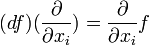$(df)(\frac{\partial}{\partial x_i}) = \frac{\partial}{\partial x_i}f$

However,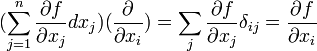$(\sum_{j=1}^{n}\frac{\partial f}{\partial x_j} dx_j)(\frac{\partial}{\partial x_i}) = \sum_j \frac{\partial f}{\partial x_j}\delta_{ij} = \frac{\partial f}{\partial x_i}$ which is the same.

Returning, we thus get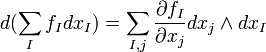$d(\sum_I f_I dx_I) = \sum_{I,j} \frac{\partial f_I}{\partial x_j} dx_j\wedge dx_I$

Thus our d takes functions to vector fields by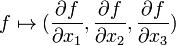$f\mapsto (\frac{\partial f}{\partial x_1},\frac{\partial f}{\partial x_2},\frac{\partial f}{\partial x_3})$

This is just the grad operator from calculus and we can see that the d operator appropriately takes things from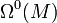$\Omega^0(M)$ to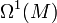$\Omega^1(M)$.

Now let us compute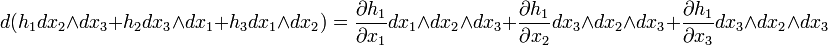$d(h_1 dx_2\wedge dx_3+h_2 dx_3\wedge dx_1+h_3 dx_1\wedge dx_2) =\frac{\partial h_1}{\partial x_1} dx_1\wedge dx_2\wedge dx_3 + \frac{\partial h_1}{\partial x_2} dx_3\wedge dx_2\wedge dx_3 + \frac{\partial h_1}{\partial x_3} dx_3\wedge dx_2\wedge dx_3$ + 6 more terms representing the 3 partials of each of the last 2 terms.

As each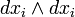$dx_i\wedge dx_i$ term vanishes we are left with just,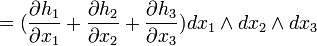$= (\frac{\partial h_1}{\partial x_1} + \frac{\partial h_2}{\partial x_2} + \frac{\partial h_3}{\partial x_3})dx_1\wedge dx_2\wedge dx_3$

I.e., d takes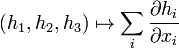$(h_1,h_2,h_3)\mapsto \sum_i\frac{\partial h_i}{\partial x_i}$

this is just the div operator from calculus and appropriately takes vector fields to functions and represents the d from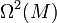$\Omega^2(M)$ to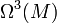$\Omega^3(M)$.

We are left with computing d from$\Omega^1(M)$ to$\Omega^2(M)$

Computing,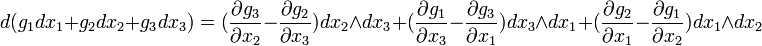$d(g_1 dx_1 + g_2 dx_2 + g_3 dx_3) = (\frac{\partial g_3}{\partial x_2} - \frac{\partial g_2}{\partial x_3})dx_2\wedge dx_3 + (\frac{\partial g_1}{\partial x_3} - \frac{\partial g_3}{\partial x_1})dx_3\wedge dx_1 + (\frac{\partial g_2}{\partial x_1} - \frac{\partial g_1}{\partial x_2})dx_1\wedge dx_2$

I.e., we just have the curl operator.

Note that the well known calculus laws that curl grad = 0 and div curl = 0 are just the expression that$d^2 =0$.

To provide some physical insight to the meanings of these operators:

1) The gradient represents the direction of maximum descent. I.e. if you had a function on the plane the graph would look like the surface of a mountain range and the direction that water would run would be the gradient.

2) In a say compressible fluid, the divergence corresponds to the difference between in the inflow and outflow of fluid in some small epsilon box around a point.

3) The curl corresponds to the rotation vector for a ball. Ie consider a ball (of equal density to the liquid about it) going down a river. In the x_2, x_1 plane the tenancy for it to rotate clockwise would be given by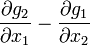$\frac{\partial g_2}{\partial x_1} - \frac{\partial g_1}{\partial x_2}$